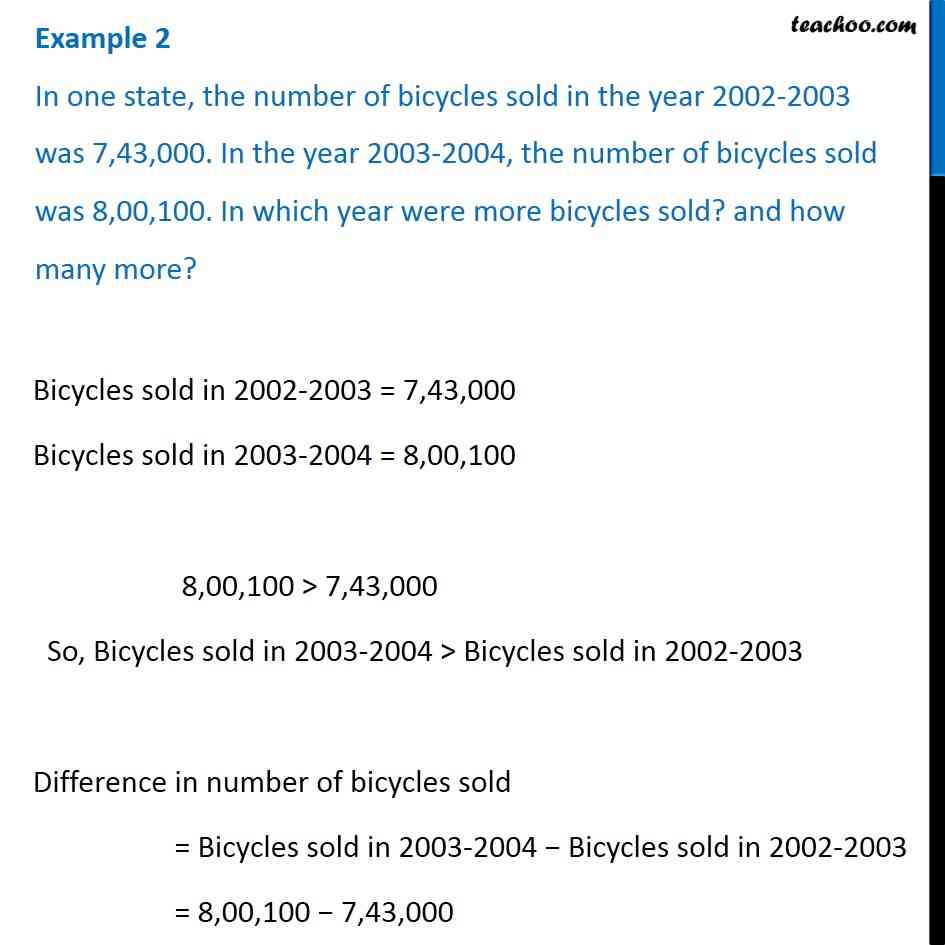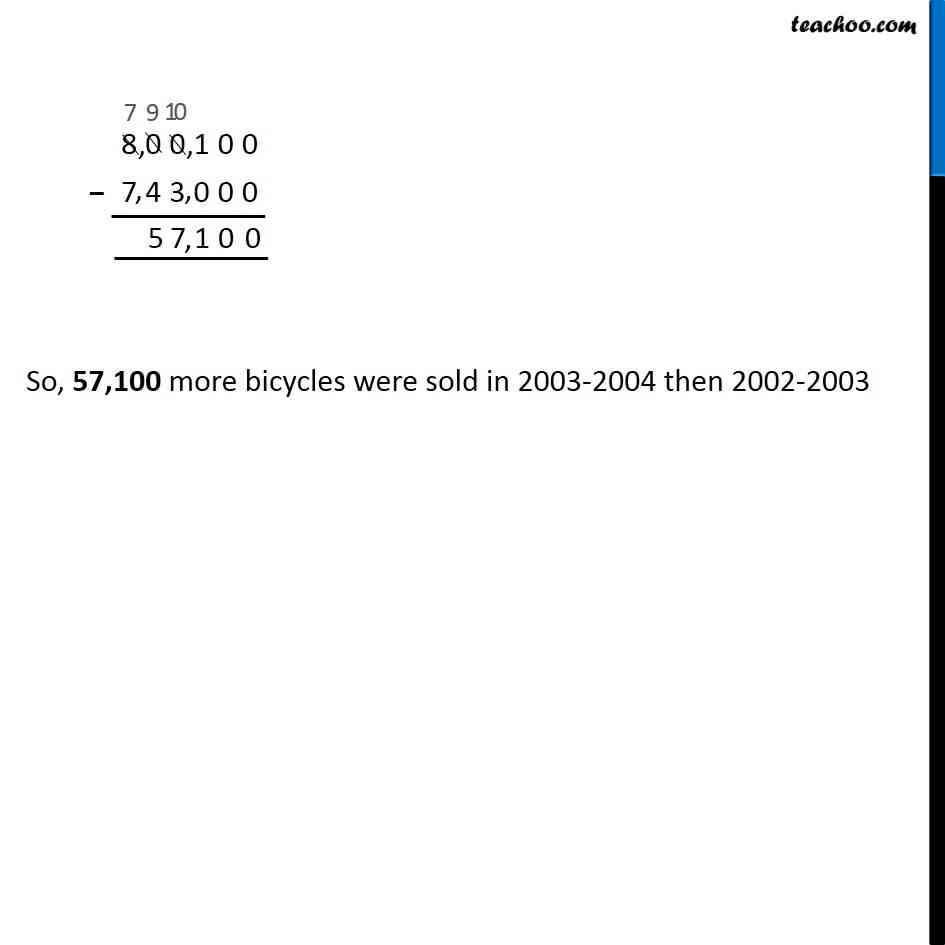1. Chapter 1 Class 6 Knowing our Numbers
2. Serial order wise
3. Examples

Transcript

Example 2 In one state, the number of bicycles sold in the year 2002-2003 was 7,43,000. In the year 2003-2004, the number of bicycles sold was 8,00,100. In which year were more bicycles sold? and how many more?Bicycles sold in 2002-2003 = 7,43,000 Bicycles sold in 2003-2004 = 8,00,100 8,00,100 > 7,43,000 So, Bicycles sold in 2003-2004 > Bicycles sold in 2002-2003 Difference in number of bicycles sold = Bicycles sold in 2003-2004 − Bicycles sold in 2002-2003 = 8,00,100 − 7,43,000

Examples